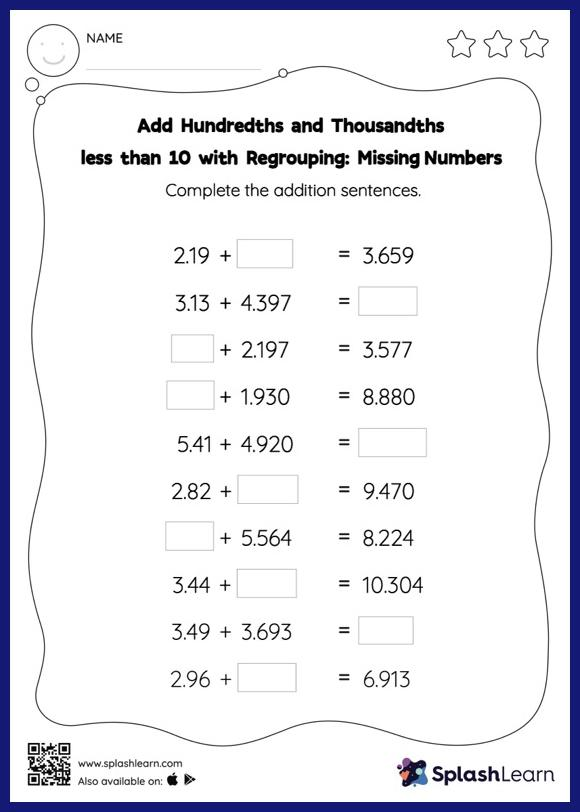# Add Hundredths and Thousandths less than 10 with Regrouping: Missing Numbers Worksheet

Home > Add Hundredths and Thousandths less than 10 with Regrouping: Missing NumbersThis add hundredths and thousandths less than 10 with regrouping worksheet consists of tasks to help your young mathematician develop fluency with addition. Students use the relationship between addition and subtraction to find the missing number while adding decimals. To reach the answer, they must also regroup the numbers in add hundredths and thousandths less than 10 with regrouping worksheet using the relationships between ones, tenths, hundredths, thousandths, etc. In each problem, the numbers are laid out in the horizontal format. Students should try to use different strategies involving composing and decomposing numbers to solve these problems. This will help them develop flexibility and fluency.